How to Learn in 24 Hours?The Rapid Learning Movie

 Need Help? M-F: 9am-5pm(PST): Toll-Free: (877) RAPID-10 US Direct: (714) 692-2900 Int'l: 001-714-692-2900 24/7 Online Technical Support: The Rapid Support Center Secure Online Order:Got Questions? Frequently Asked Questions
 Need Proof? Testimonials by Our Users
 Trustlink is a Better Business Bureau Program. Rapid Learning Center is a fivr-star business. External TrustLink Reviews

 Rapid Learning Courses: MCAT in 24 Hours (2015-16) USMLE in 24 Hours (Boards) Chemistry in 24 Hours Biology in 24 Hours Physics in 24 Hours Mathematics in 24 Hours Psychology in 24 Hours SAT in 24 Hours ACT in 24 Hours AP in 24 Hours CLEP in 24 Hours DAT in 24 Hours (Dental) OAT in 24 Hours (Optometry) PCAT in 24 Hours (Pharmacy) Nursing Entrance Exams Certification in 24 Hours eBook - Survival Kits Audiobooks (MP3)

 Tell-A-Friend: Have friends taking science and math courses too? Tell them about our rapid learning system.

 FAIL (the browser should render some flash content, not this).Home »  Mathematics »  Trigonometry

Laws of Sines and Cosines

 Topic Review on "Title": Law of Sines: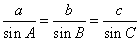= side of triangle ABC divide by the sine of angle opposite side Law of Cosines: For a triangle ABC to find, b and c we use the formula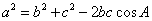. For a triangle ABC to find, a and c then we use the formula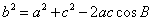. For a triangle ABC to find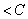, a and b then we use the formula.

Rapid Study Kit for "Title":
 Flash Movie Flash Game Flash Card Core Concept Tutorial Problem Solving Drill Review Cheat Sheet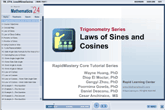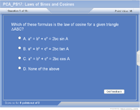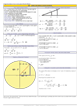"Title" Tutorial Summary : This tutorial deals with the laws of sines and cosines and their applications. A more accurate approximation of solutions can be performed by using the law of sines and cosines. Scenarios are given where some angles or sides of a triangle are not known so the law of sines and cosines are used in examples. Scenarios are given where some angles or sides of a triangle are not known so the law of sines and cosines are used in examples. Other scenarios of where a more detailed solving process are performed in the example problems Acronyms are giving for the different cases of the law of sines and the various cases of the law of cosines.

 Tutorial Features: Specific Tutorial Features: • Diagrams showing ASA, SSS and AAA sine and cosines scenarios are mentioned in this tutorial. • Illustrations showing all aspects of the law of sines and cosines. Series Features: • Concept map showing inter-connections of new concepts in this tutorial and those previously introduced. • Definition slides introduce terms as they are needed. • Visual representation of concepts • Animated examples—worked out step by step • A concise summary is given at the conclusion of the tutorial.

 "Title" Topic List: Law of Sines Cycle representation using the law of sines Side-Angle Side (SAS) and examples Angle-Side-Angle (ASA) and examples Side-Side-Angle (SSA) and examplesLaw of Cosines Side-Angle-Side (SAS) and examples Side-Side-Side (SSS) and examples

See all 24 lessons in Trigonometry, including concept tutorials, problem drills and cheat sheets:
Teach Yourself Trigonometry Visually in 24 Hours